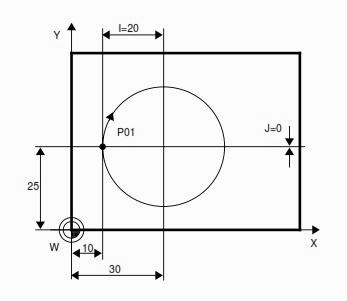# How to Mill Full Circle CNC Program Example Code

How to mill a full circle, no problem below is the cnc program example G-code which shows how to program a full circle on a cnc milling machine.

Often cnc machinists has to complete such components which require a full circle machining sometimes inside full circle sometimes you have to program an outside full circle.

This G-code example will show you how to program a full circle from out-side.

## Full Circle CNC Program ExampleHow to Mill Full Circle CNC Program

```N05 G00 X10 Y25 Z1 S1250 M3
N10 G01 Z-5 F100
N15 G02 X10 Y25 I20 J0 F125
N20 G00 Z100 M5
N25 X-20
N30 M30```

## How to Program Full Circle Explanation

### Summary

N05 Tool rapid traverse (G00) to point P01.
N10 Infeed (G01) to Z-5
N15 X-Y plane selected automatically (G17). Tool travels clockwise around a full circle (G02).
N20/N25 Rapid traverse (G00) retraction.
N30 End of program (M30).

### Explanation

1: The tool start point is N05 X10 Y25 this is the point P01

2: Now we want a complete circle so we will use G02 or G03 circular interpolation G-code
If you want a clock-wise circle you will use G02 as shown in this program.
but if you want a counter-clock-wise circle then use G03 G-code.

3: With G02 we will give the coordinates of the circle end point,
as for a full circle the start-point and end-point remains the same,
so we will use same X,Y coordinate
G02 X10 Y25

4: Now to complete G02 G-code we will have to give the circle-center-point coordinates,
I – Distance from circle start-point to circle-center-point in X-axis.
J – Distance from circle start-point to circle-center-point in Y-axis
so G02 X10 Y25 I20 J0
to show how to measure I and J the values of I and  J are separately given in the above figure.